# Practical Geometry Class 8 Notes- Chapter 4

According to the CBSE Syllabus 2023-24, this chapter has been removed from NCERT Class 8 Maths textbook.

## CBSE Practical Geometry Class 8 Notes – Chapter 4:-

Class 8 Practical Geometry chapter will teach you to construct quadrilaterals given different kinds of measurements. A quadrilateral is a closed two-dimensional shape that has four sides and four angles. Any four-sided closed shape such as square, rectangle, rhombus, parallelogram, trapezium, etc., is a quadrilateral. Let us learn here how to construct a quadrilateral given its five measurements.

## Introduction to Practical Geometry

#### For more information on Introduction to Practical Geometry, watch the below video.### Number of measurements necessary for construction of a unique Quadrilateral

To draw a unique quadrilateral we need at least five measurements of sides and anglesHowever, it is not necessary that we will get a unique quadrilateral if we have the measurements of any five combinations of sides and angles.

For example, a unique quadrilateral can be drawn if we are given the measurement of four sides and one diagonal of a quadrilateral.
However, a unique quadrilateral will not be drawn if we are given the measurement of two diagonals and three angles of a quadrilateral.

It is very easy to construct a quadrilateral when its five measurements are determined that is

• The length of the four sides and the length of its diagonal is known
• The length of the three sides and the length of the two diagonals are known
• If the three angles and two adjacent sides are given
• If the three sides and two angles are given

## 4 Sides and 1 Diagonal

### Construction of a Quadrilateral when different measures of sides and angles are given

A unique quadrilateral can be constructed when the following measurements are given:

• Four sides and one diagonal.
• Two diagonals and three sides.
• Two adjacent sides and three angles.
• Three sides and two included angles.
• When other special properties are known.

### SSS Construction

To construct a ABC, the length of whose sides are, AB = x cm, BC = y cm, and AC = z cm, we will do it in the following manner:

• Step 1: Construct a line segment AB, whose length is x cm.
• Step 2: With A as the center, draw an arc of radius z cm.
• Step 3: With B as the center, draw an arc of radius y cm on the same side. The point where the arcs intersect is the required point C.
• Step 4: Join AC and BC.

ABC is the required triangle.

### Construction of a Quadrilateral when four sides and one diagonal are given

Suppose we have to construct a quadrilateral PQRS, where PQ = 4 cm, QR = 6 cm, RS = 5 cm, PS = 5.5 cm and PR = 7 cm.
Step 1: Draw a rough sketch to visualize the quadrilateral.Step 2: Draw PQR as it can be constructed using SSS construction condition.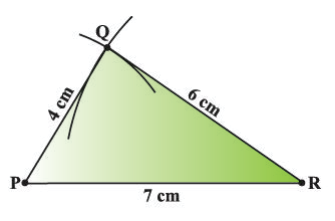Step 3: Now we have to locate S, which is at a distance of 5.5 cm from P and 5 cm from R. Also it will be on the opposite side of Q.
With P as center draw an arc of radius 5.5 cm. With R as center draw an arc of radius 5 cm.
S is the point of intersection of the two arcs.

Step 4: Join PS and RS. PQRS is the required quadrilateral.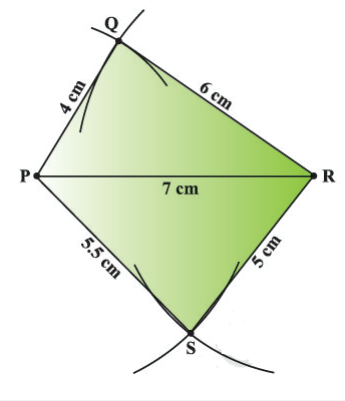Know more: Constructing Triangle with SSS Congruence

## 3 Sides and 2 Diagonals

### Construction of a Quadrilateral when two diagonals and three sides are given

Construct a quadrilateral ABCD given, AB = 7 cm, AD = 6 cm, AC = 7 cm, BD = 7.5 cm and BC = 4 cm.
[Make a rough figure for your reference]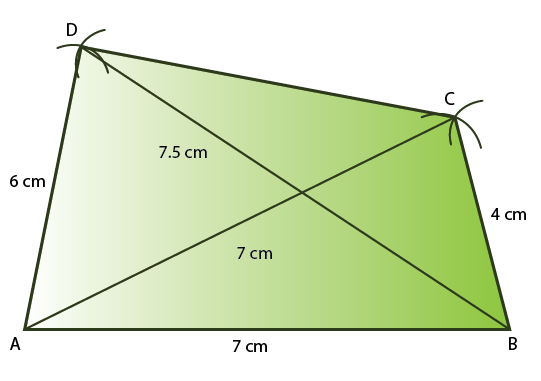Steps of construction of the quadrilateral:

Step 1: ABC can be drawn by SSS construction condition since all its sides are known.
Step 2: With A as center and radius 6 cm (AD), draw an arc.
Step 3: With B as center and radius 7.5 cm (BD) draw another arc to cut the previous arc at D
Step 4: Join AD, BD, and CD.

## 2 Adjacent Sides and 3 Angles

### Construction of a Quadrilateral when two adjacent sides and three angles are given

Construct a quadrilateral ALPN, where AL = 6.5 cm, LP = 4 cm, NAL = 110ALP = 75 and LPN = 90.
[Draw a rough Sketch for your reference]:
Steps of construction of the quadrilateral:
Step 1: Draw the line segment AL of length 6.5 cm.
Step 2: Make ALY = 75 at L.
Step 3: Make LAX = 110 at A.
Step 4: With L as center and radius equal to 4 cm, cut an arc on the ray LY at P.
Step 5: Make LPZ = 90 at P.
Step 6: Name the point of intersection of rays PZ and AX as N.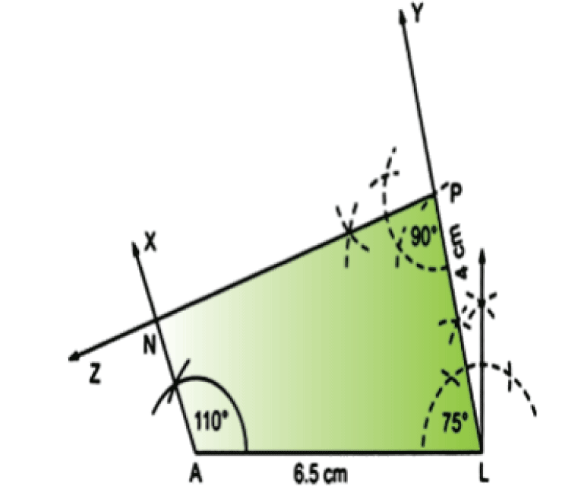## 3 Sides and 2 Included Angles

### Construction of a Quadrilateral when three Sides and two included angles are given

Construct a quadrilateral ABCD, Where AB = 4.5 cm; BC = 3.5 cm, CD = 5 cm ABC = 45, BCD = 150
[Make a rough figure for your reference]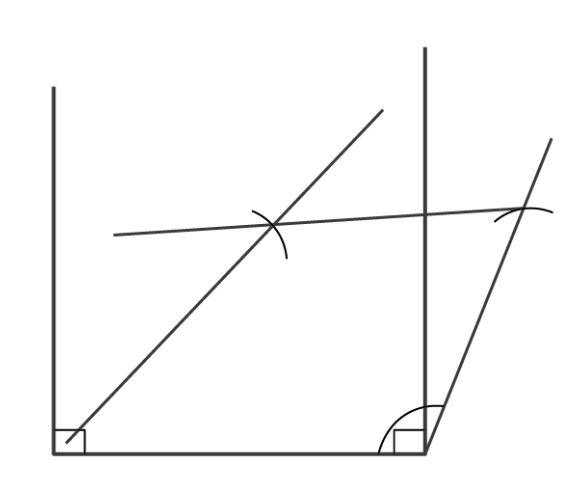Steps of construction of the quadrilateral:
Step 1: Draw a line segment BC of length 3.5 cm.
Step 2: Make LBC =  45.
Step 3: Make BCM = 150.
Step 4: With B as center and radius equal to 4.5 cm, cut an arc on the ray LB at A.
Step 5: With C as the center and radius equal to 5 cm, cut an arc on the ray CM at D.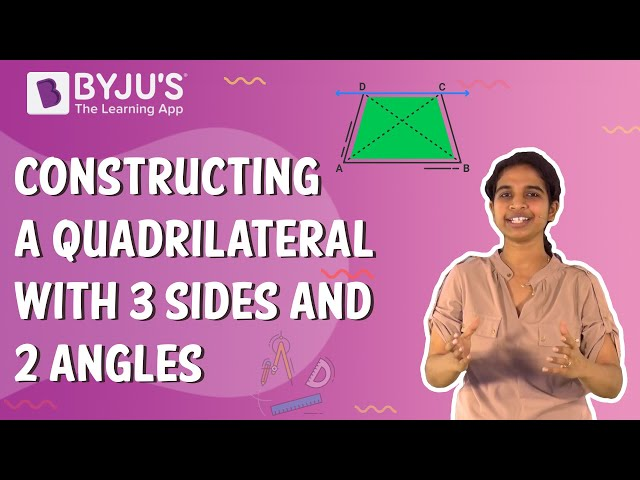### Construction of a Quadrilateral When Other Special Properties Are Known

Construct a rhombus PQRS with diagonals PR = 5.2 cm and QS = 6.4 cm
[Make a rough figure for your reference]

Note: Diagonals of a rhombus are perpendicular bisectors of each other.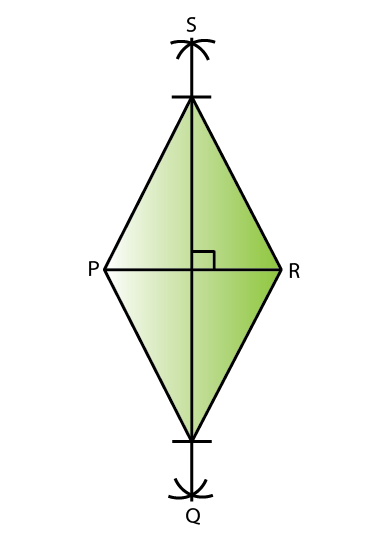Steps of construction of the Rhombus:
Step 1: Draw a line segment PR of length 5.2 cm.
Step 2: Draw the perpendicular bisector of PR. Name the point O, where the perpendicular bisector of PR and PR intersect.
Step 3: With O as center and radius equal to 3.2 cm cut arcs on both sides of the perpendicular bisector. Name them as Q and S.
Step 4: Join, PQ, QR, RS, and PS.

PQRS is the required rhombus.
Know more: Construction of Rhombus

## Frequently asked Questions on CBSE Class 8 Maths Notes Chapter 4 Practical Geometry

Q1

### What are some of the uses of practical geometry?

1. Computer graphics 2. Construction of buildings 3. Art 4. Architecture and interior designing 5. Study of orbits and planetary motions

Q2

### What is a Line segment?

In geometry, a line segment is a part of a line that is bounded by two distinct end points and contains every point on the line that is between its endpoints.

Q3

### How can a circle be defined?

A circle consists of a closed curved line around a central point. Every point on the line is the same distance from the central point.